# NCERT Exemplar Class 11 Chemistry Solutions for Chapter 2 - Structure of Atom

## NCERT Exemplar Solutions Class 11 Chemistry Chapter 2 – Free PDF Download

NCERT Exemplar Chemistry Class 11 Chapter 2 Structure of Atom is the best study material you can rely upon to prepare for CBSE Class 11 and entrance exam preparation. Studying this exemplar solution will help you in grasping the concepts deeply and you can easily take out chemistry Chapter 2 Class 11 notes to help you in revising the chapter. NCERT Exemplar Class 11 Chemistry Chapter 2 has questions from the NCERT exemplar book, questions from previous year question papers, the structure of atom Class 11 numerical, exercises, worksheets, tips, and tricks to help you excel in CBSE Class11 examinations. The exemplar contains well-written answers for all types of questions given at the end of the chapter. This will further enable students to complete assignments and to tackle any type of question that can be asked in the exams.

When students study Chapter 2, they will basically be learning about the experimental observations made by scientists in the field of Chemistry. Students will also get to know about how electron, proton, and neutron were discovered and learn their characteristics. They will also be introduced to concepts like Thomson, Rutherford, and Bohr atomic models. While the chapter needs more attention students should understand each topic clearly. We are providing free NCERT Exemplar for Class 11 Chemistry Chapter 2 – the structure of atom here. The NCERT Exemplar can be easily downloaded in the form of a PDF and has been designed to help the students to have productive study time.

### Download PDF of NCERT Exemplar for Class 11 Chemistry Chapter 2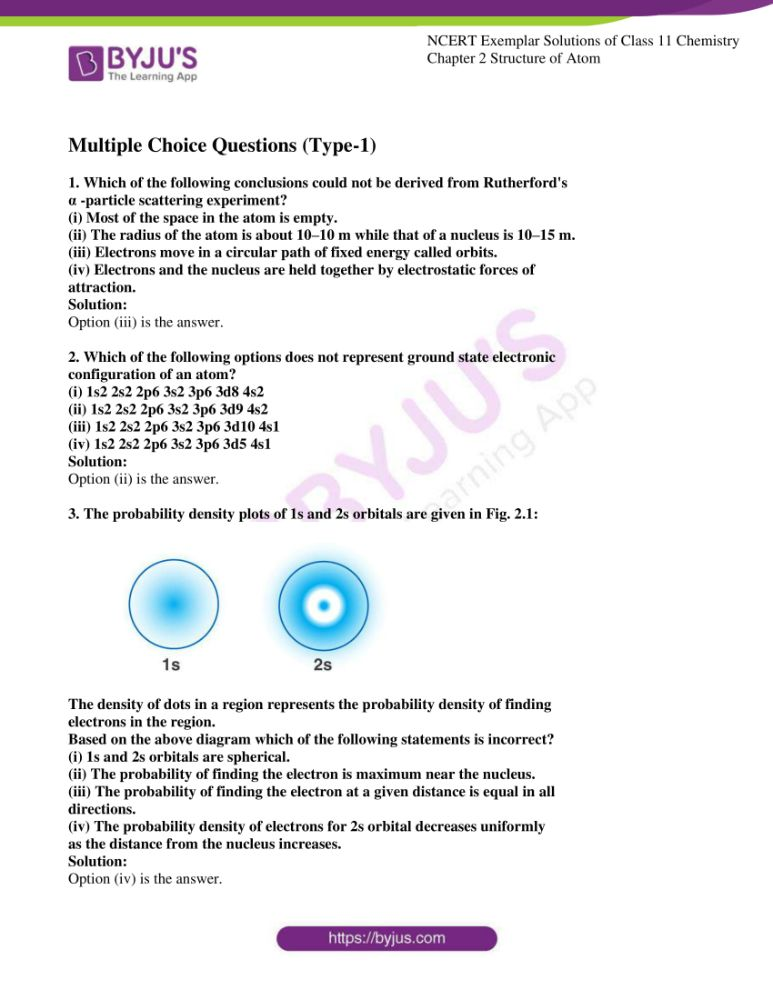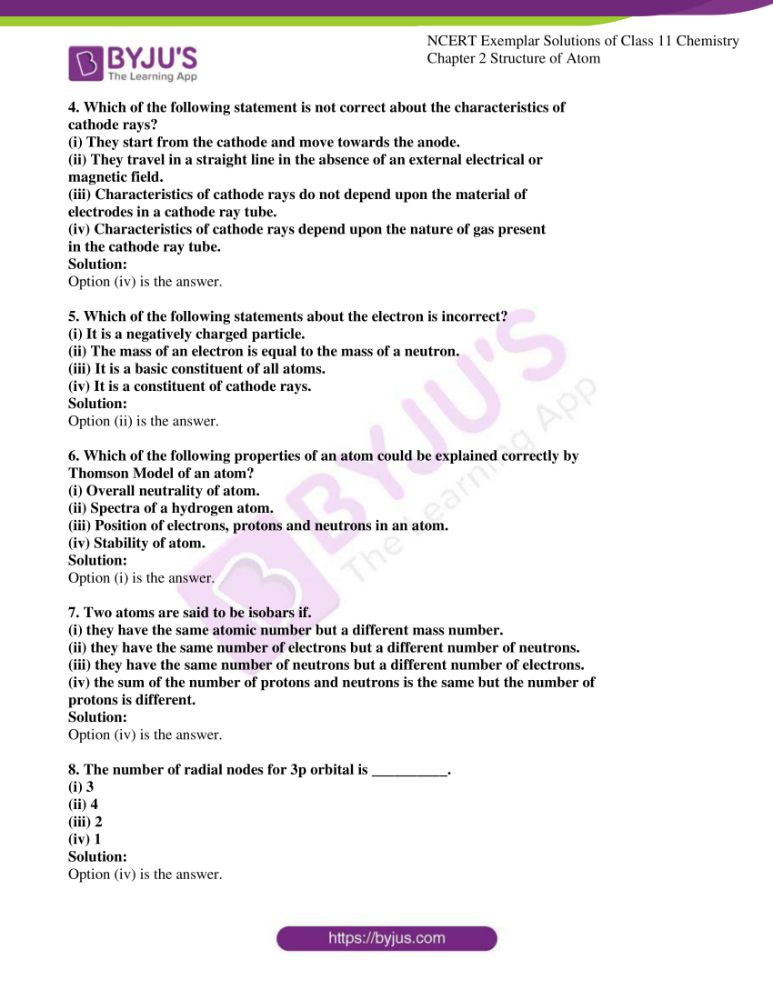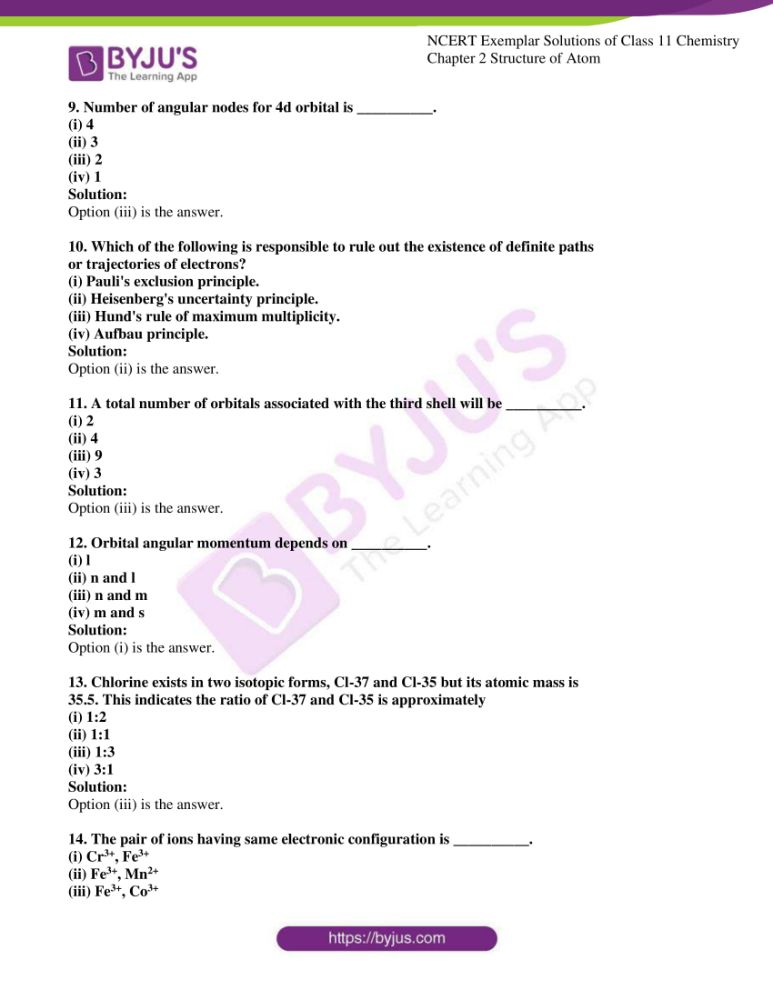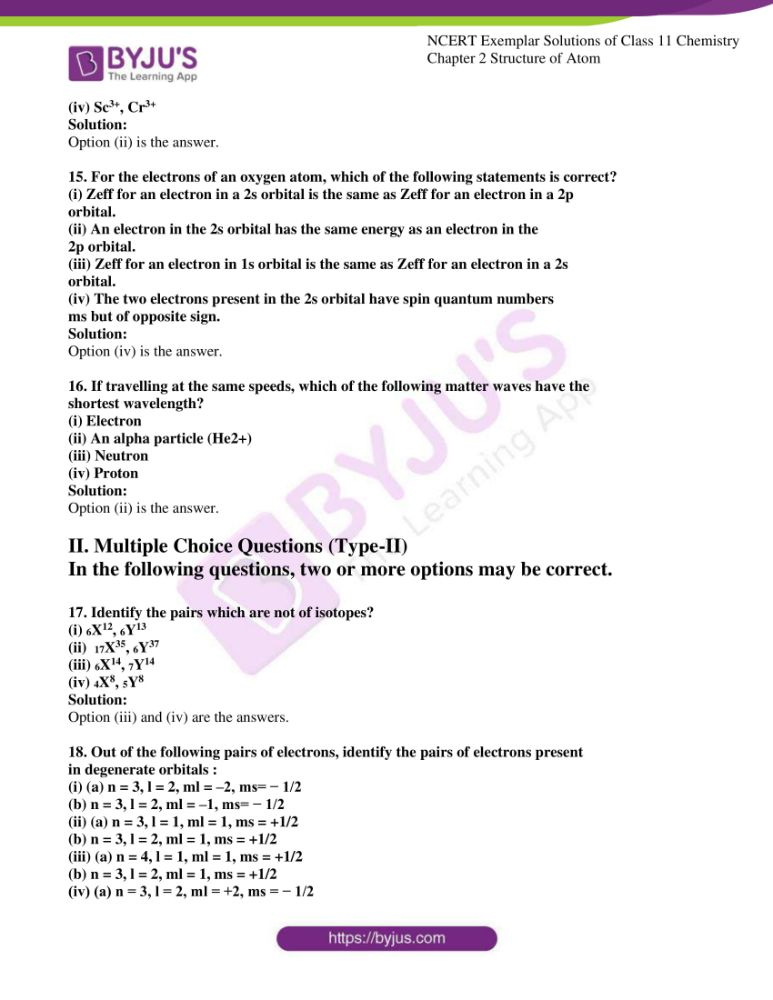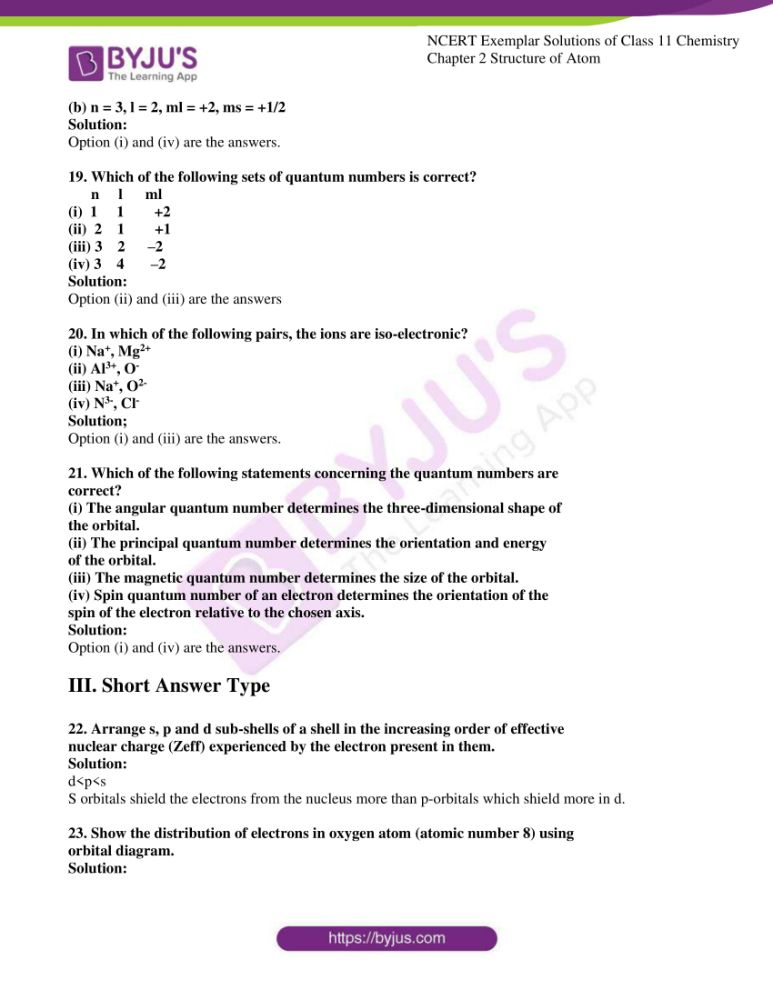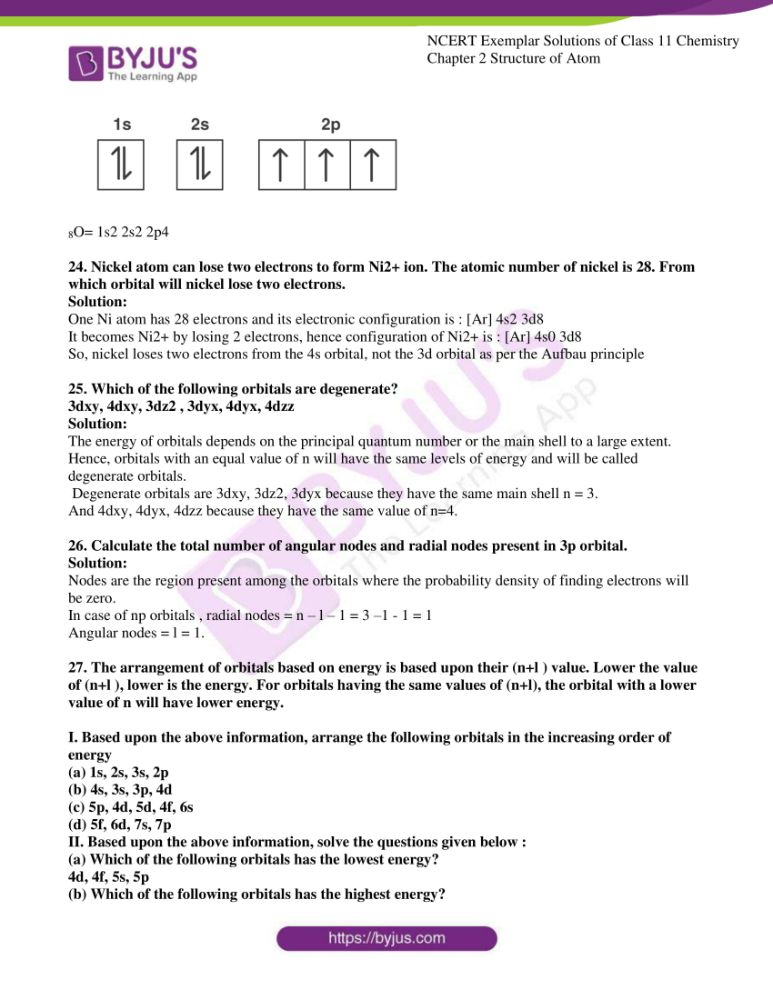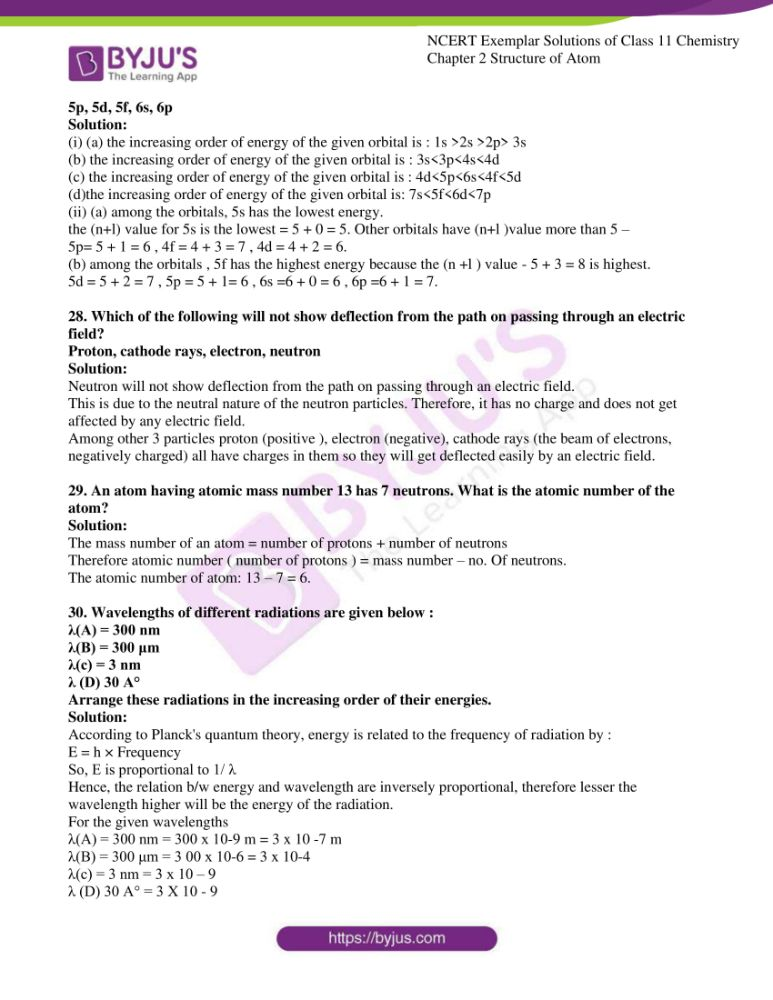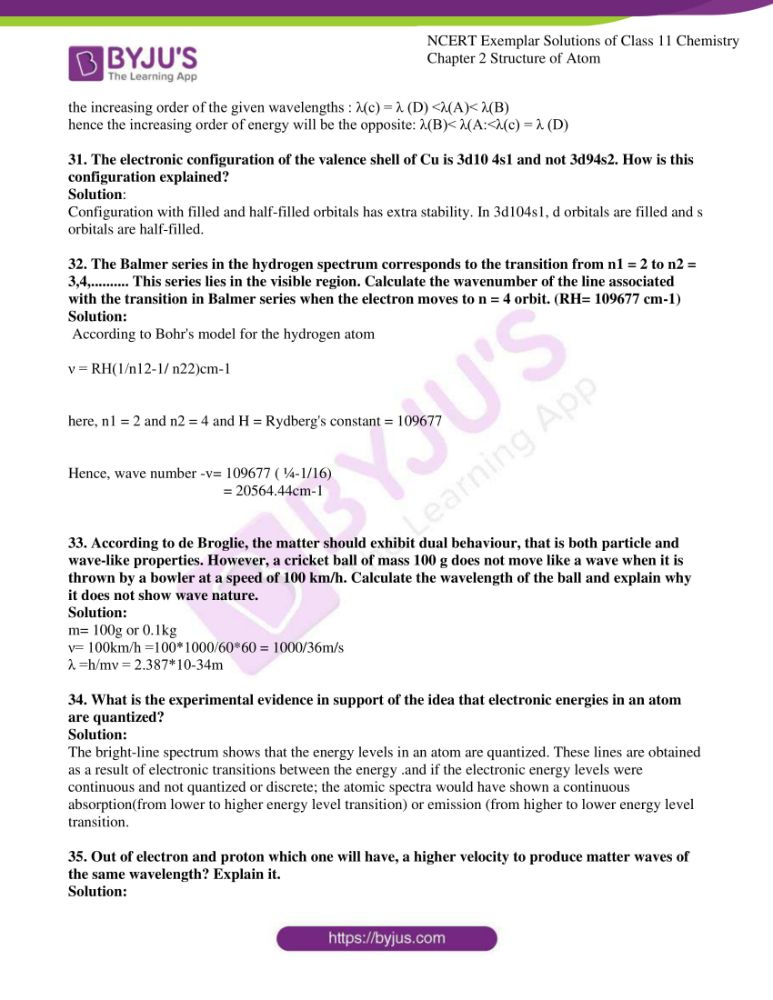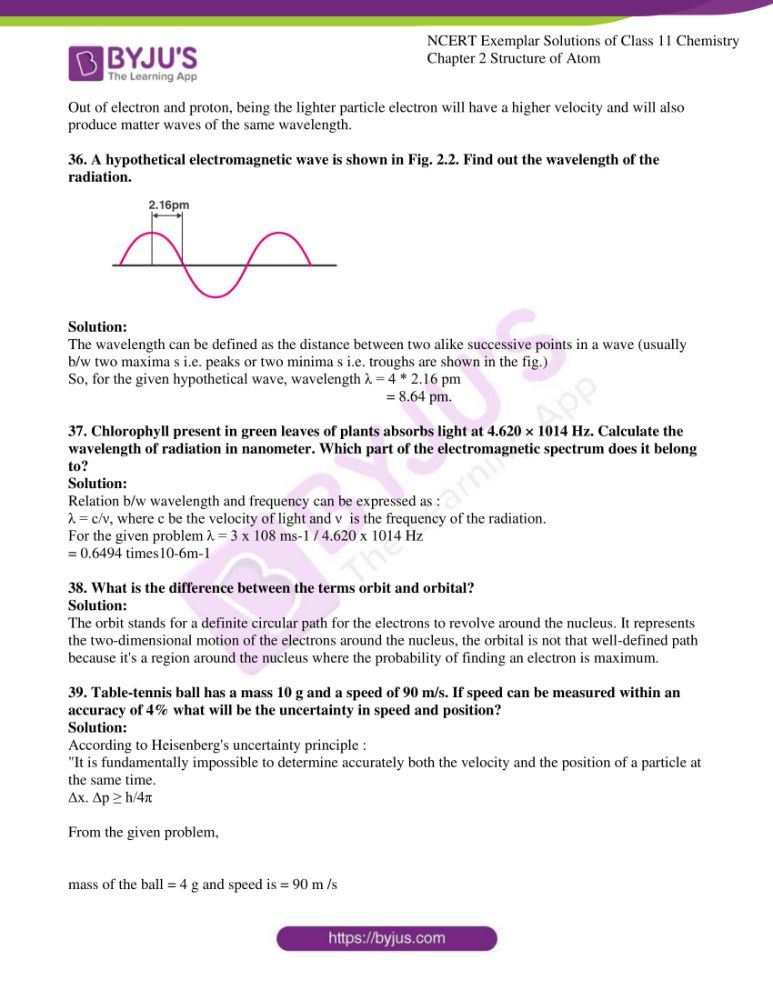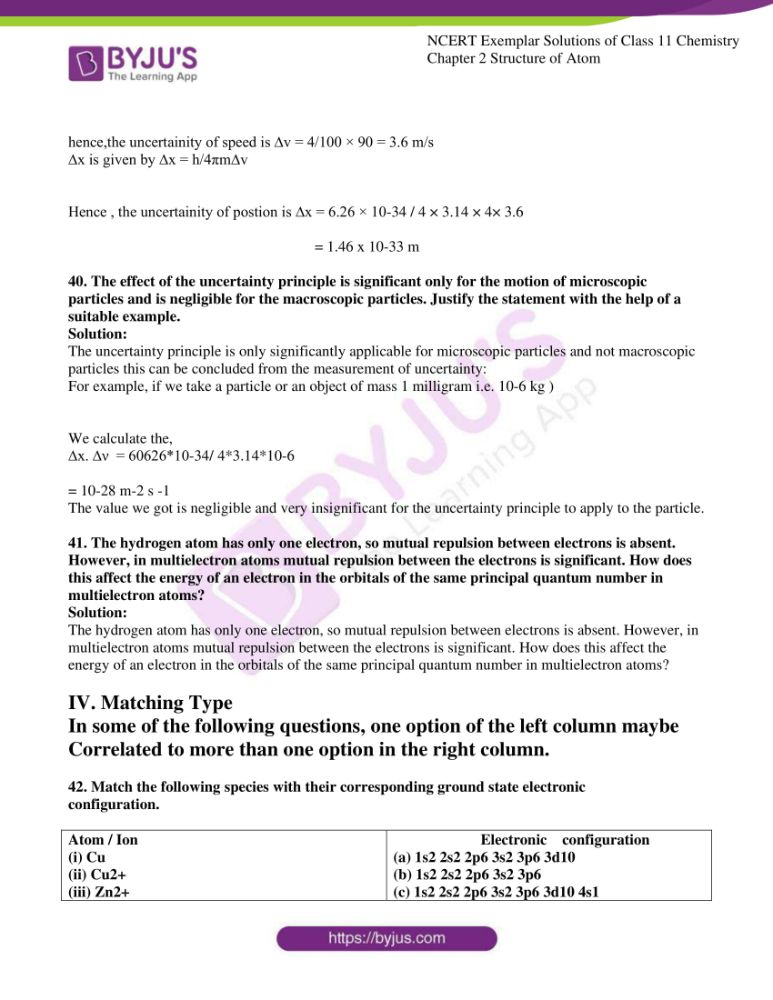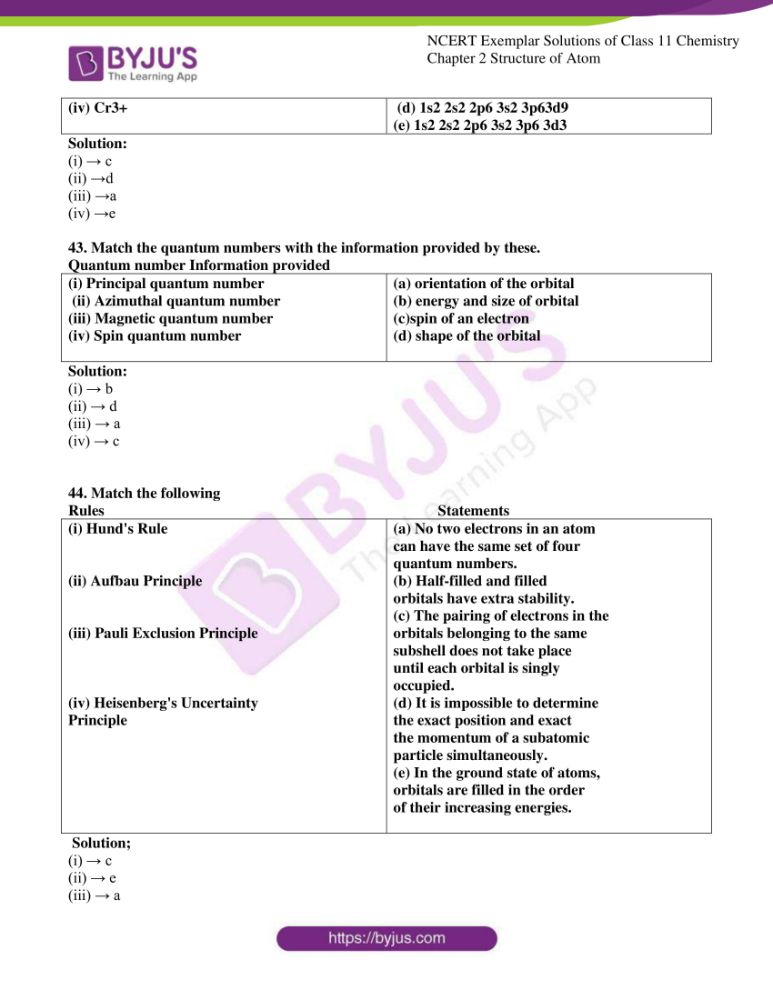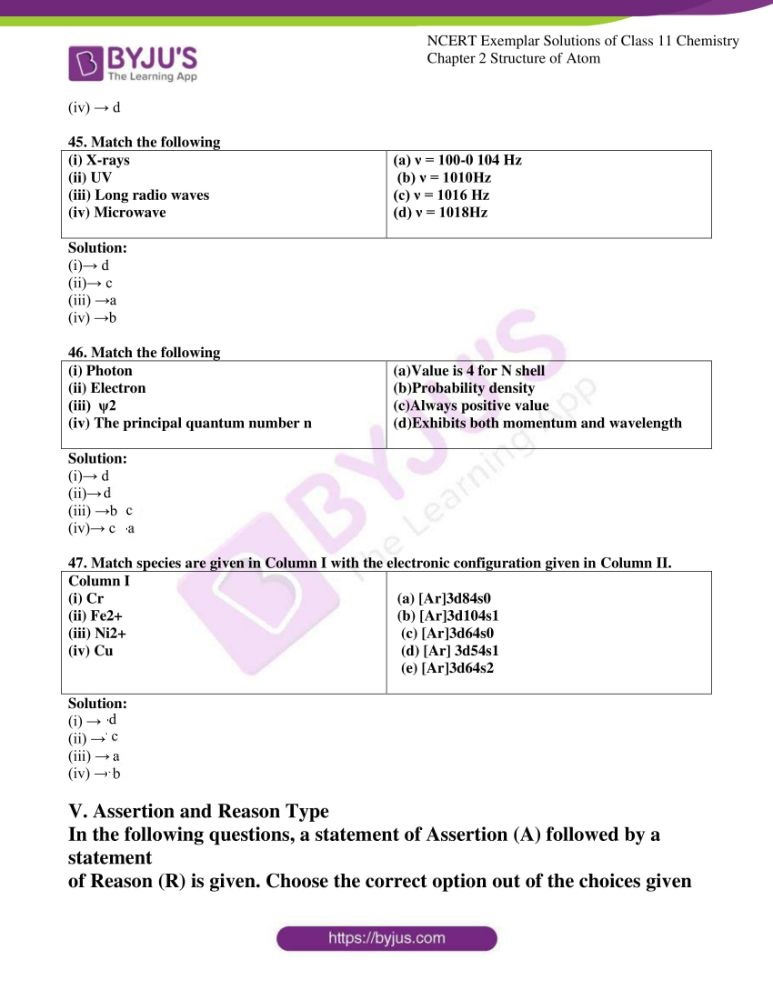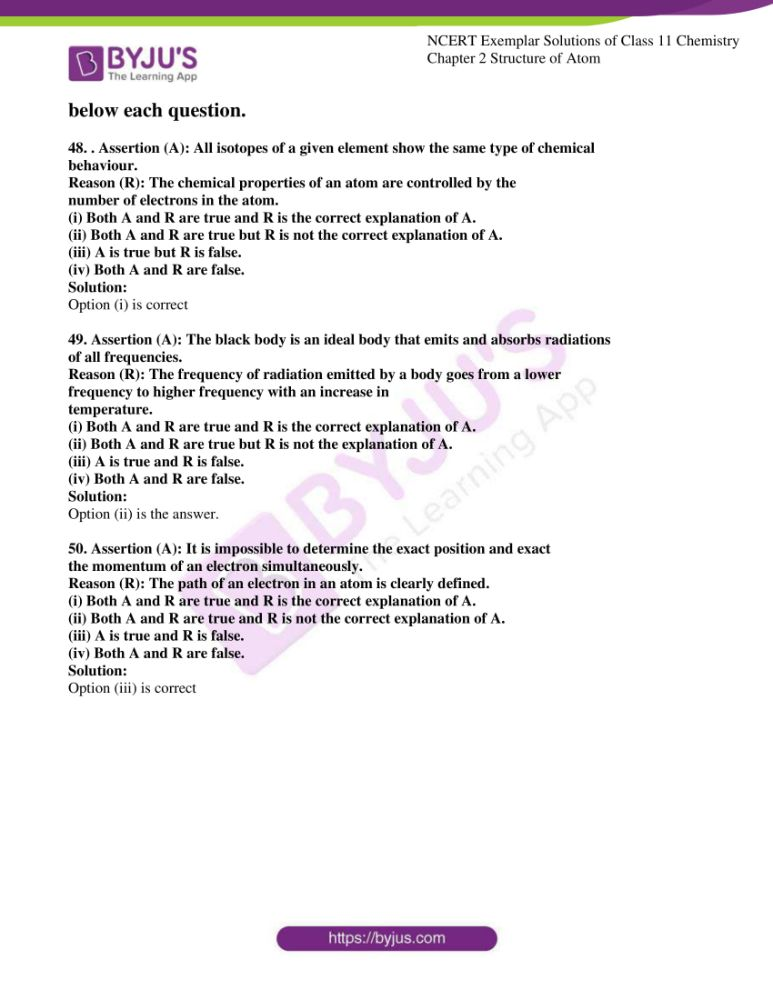### Access Answers to NCERT Exemplar Class 11 Chemistry Chapter 2

Multiple Choice Questions (Type-1)

1. Which of the following conclusions could not be derived from Rutherford’s

α -particle scattering experiment?

(i) Most of the space in the atom is empty.

(ii) The radius of the atom is about 10–10 m while that of a nucleus is 10–15 m.

(iii) Electrons move in a circular path of fixed energy called orbits.

(iv) Electrons and the nucleus are held together by electrostatic forces of

attraction.

Solution:

2. Which of the following options does not represent ground state electronic

configuration of an atom?

(i) 1s2 2s2 2p6 3s2 3p6 3d8 4s2

(ii) 1s2 2s2 2p6 3s2 3p6 3d9 4s2

(iii) 1s2 2s2 2p6 3s2 3p6 3d10 4s1

(iv) 1s2 2s2 2p6 3s2 3p6 3d5 4s1

Solution:

3. The probability density plots of 1s and 2s orbitals are given in Fig. 2.1: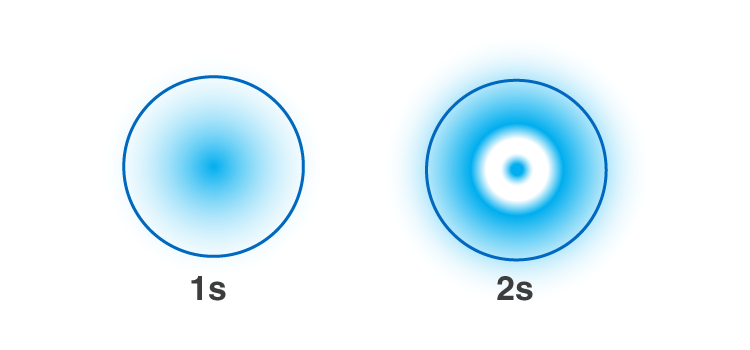The density of dots in a region represents the probability density of finding

electrons in the region.

Based on the above diagram which of the following statements is incorrect?

(i) 1s and 2s orbitals are spherical.

(ii) The probability of finding the electron is maximum near the nucleus.

(iii) The probability of finding the electron at a given distance is equal in all

directions.

(iv) The probability density of electrons for 2s orbital decreases uniformly

as the distance from the nucleus increases.

Solution:

4. Which of the following statement is not correct about the characteristics of

cathode rays?

(i) They start from the cathode and move towards the anode.

(ii) They travel in a straight line in the absence of an external electrical or

magnetic field.

(iii) Characteristics of cathode rays do not depend upon the material of

electrodes in a cathode ray tube.

(iv) Characteristics of cathode rays depend upon the nature of gas present

in the cathode ray tube.

Solution:

5. Which of the following statements about the electron is incorrect?

(i) It is a negatively charged particle.

(ii) The mass of an electron is equal to the mass of a neutron.

(iii) It is a basic constituent of all atoms.

(iv) It is a constituent of cathode rays.

Solution:

6. Which of the following properties of an atom could be explained correctly by

Thomson Model of an atom?

(i) Overall neutrality of atom.

(ii) Spectra of a hydrogen atom.

(iii) Position of electrons, protons and neutrons in an atom.

(iv) Stability of atom.

Solution:

7. Two atoms are said to be isobars if.

(i) they have the same atomic number but a different mass number.

(ii) they have the same number of electrons but a different number of neutrons.

(iii) they have the same number of neutrons but a different number of electrons.

(iv) the sum of the number of protons and neutrons is the same but the number of

protons is different.

Solution:

8. The number of radial nodes for 3p orbital is __________.

(i) 3

(ii) 4

(iii) 2

(iv) 1

Solution:

9. Number of angular nodes for 4d orbital is __________.

(i) 4

(ii) 3

(iii) 2

(iv) 1

Solution:

10. Which of the following is responsible to rule out the existence of definite paths

or trajectories of electrons?

(i) Pauli’s exclusion principle.

(ii) Heisenberg’s uncertainty principle.

(iii) Hund’s rule of maximum multiplicity.

(iv) Aufbau principle.

Solution:

11. A total number of orbitals associated with the third shell will be __________.

(i) 2

(ii) 4

(iii) 9

(iv) 3

Solution:

12. Orbital angular momentum depends on __________.

(i) l

(ii) n and l

(iii) n and m

(iv) m and s

Solution:

13. Chlorine exists in two isotopic forms, Cl-37 and Cl-35 but its atomic mass is

35.5. This indicates the ratio of Cl-37 and Cl-35 is approximately

(i) 1:2

(ii) 1:1

(iii) 1:3

(iv) 3:1

Solution:

14. The pair of ions having same electronic configuration is __________.

(i) Cr3+, Fe3+

(ii) Fe3+, Mn2+

(iii) Fe3+, Co3+

(iv) Sc3+, Cr3+

Solution:

15. For the electrons of an oxygen atom, which of the following statements is correct?

(i) Zeff for an electron in a 2s orbital is the same as Zeff for an electron in a 2p

orbital.

(ii) An electron in the 2s orbital has the same energy as an electron in the

2p orbital.

(iii) Zeff for an electron in 1s orbital is the same as Zeff for an electron in a 2s

orbital.

(iv) The two electrons present in the 2s orbital have spin quantum numbers

ms but of opposite sign.

Solution:

16. If travelling at the same speeds, which of the following matter waves have the

shortest wavelength?

(i) Electron

(ii) An alpha particle (He2+)

(iii) Neutron

(iv) Proton

Solution:

II. Multiple Choice Questions (Type-II)

In the following questions, two or more options may be correct.

17. Identify the pairs which are not of isotopes?

(i) 6X12, 6Y13

(ii) 17X35, 6Y37

(iii) 6X14, 7Y14

(iv) 4X8, 5Y8

Solution:

Option (iii) and (iv) are the answers.

18. Out of the following pairs of electrons, identify the pairs of electrons present

in degenerate orbitals :

(i) (a) n = 3, l = 2, ml = –2, ms= − 1/2

(b) n = 3, l = 2, ml = –1, ms= − 1/2

(ii) (a) n = 3, l = 1, ml = 1, ms = +1/2

(b) n = 3, l = 2, ml = 1, ms = +1/2

(iii) (a) n = 4, l = 1, ml = 1, ms = +1/2

(b) n = 3, l = 2, ml = 1, ms = +1/2

(iv) (a) n = 3, l = 2, ml = +2, ms = − 1/2

(b) n = 3, l = 2, ml = +2, ms = +1/2

Solution:

Option (i) and (iv) are the answers.

19. Which of the following sets of quantum numbers is correct?

n l ml

(i) 1 1 +2

(ii) 2 1 +1

(iii) 3 2 –2

(iv) 3 4 –2

Solution:

Option (ii) and (iii) are the answers

20. In which of the following pairs, the ions are iso-electronic?

(i) Na+, Mg2+

(ii) Al3+, O

(iii) Na+, O2-

(iv) N3-, Cl

Solution;

Option (i) and (iii) are the answers.

21. Which of the following statements concerning the quantum numbers are

correct?

(i) The angular quantum number determines the three-dimensional shape of

the orbital.

(ii) The principal quantum number determines the orientation and energy

of the orbital.

(iii) The magnetic quantum number determines the size of the orbital.

(iv) Spin quantum number of an electron determines the orientation of the

spin of the electron relative to the chosen axis.

Solution:

Option (i) and (iv) are the answers.

22. Arrange s, p and d sub-shells of a shell in the increasing order of effective

nuclear charge (Zeff) experienced by the electron present in them.

Solution:

d<p<s

S orbitals shield the electrons from the nucleus more than p-orbitals which shield more in d.

23. Show the distribution of electrons in oxygen atom (atomic number 8) using

orbital diagram.

Solution: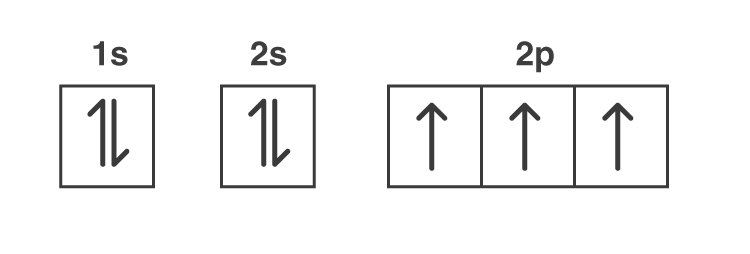8O= 1s2 2s2 2p4

24. Nickel atom can lose two electrons to form Ni2+ ion. The atomic number of nickel is 28. From which orbital will nickel lose two electrons.

Solution:

One Ni atom has 28 electrons and its electronic configuration is : [Ar] 4s2 3d8

It becomes Ni2+ by losing 2 electrons, hence configuration of Ni2+ is : [Ar] 4s0 3d8

So, nickel loses two electrons from the 4s orbital, not the 3d orbital as per the Aufbau principle

25. Which of the following orbitals are degenerate?

3dxy, 4dxy, 3dz2 , 3dyx, 4dyx, 4dzz

Solution:

The energy of orbitals depends on the principal quantum number or the main shell to a large extent. Hence, orbitals with an equal value of n will have the same levels of energy and will be called degenerate orbitals.

Degenerate orbitals are 3dxy, 3dz2, 3dyx because they have the same main shell n = 3.

And 4dxy, 4dyx, 4dzz because they have the same value of n=4.

26. Calculate the total number of angular nodes and radial nodes present in 3p orbital.

Solution:

Nodes are the region present among the orbitals where the probability density of finding electrons will be zero.

In case of np orbitals , radial nodes = n – l – 1 = 3 –1 – 1 = 1

Angular nodes = l = 1.

27. The arrangement of orbitals based on energy is based upon their (n+l ) value. Lower the value of (n+l ), lower is the energy. For orbitals having the same values of (n+l), the orbital with a lower value of n will have lower energy.

I. Based upon the above information, arrange the following orbitals in the increasing order of energy

(a) 1s, 2s, 3s, 2p

(b) 4s, 3s, 3p, 4d

(c) 5p, 4d, 5d, 4f, 6s

(d) 5f, 6d, 7s, 7p

II. Based upon the above information, solve the questions given below :

(a) Which of the following orbitals has the lowest energy?

4d, 4f, 5s, 5p

(b) Which of the following orbitals has the highest energy?

5p, 5d, 5f, 6s, 6p

Solution:

(i) (a) the increasing order of energy of the given orbital is : 1s >2s >2p> 3s

(b) the increasing order of energy of the given orbital is : 3s<3p<4s<4d

(c) the increasing order of energy of the given orbital is : 4d<5p<6s<4f<5d

(d)the increasing order of energy of the given orbital is: 7s<5f<6d<7p

(ii) (a) among the orbitals, 5s has the lowest energy.

the (n+l) value for 5s is the lowest = 5 + 0 = 5. Other orbitals have (n+l )value more than 5 –

5p= 5 + 1 = 6 , 4f = 4 + 3 = 7 , 4d = 4 + 2 = 6.

(b) among the orbitals , 5f has the highest energy because the (n +l ) value – 5 + 3 = 8 is highest.

5d = 5 + 2 = 7 , 5p = 5 + 1= 6 , 6s =6 + 0 = 6 , 6p =6 + 1 = 7.

28. Which of the following will not show deflection from the path on passing through an electric field?

Proton, cathode rays, electron, neutron

Solution:

Neutron will not show deflection from the path on passing through an electric field.

This is due to the neutral nature of the neutron particles. Therefore, it has no charge and does not get affected by any electric field.

Among other 3 particles proton (positive ), electron (negative), cathode rays (the beam of electrons, negatively charged) all have charges in them so they will get deflected easily by an electric field.

29. An atom having atomic mass number 13 has 7 neutrons. What is the atomic number of the atom?

Solution:

The mass number of an atom = number of protons + number of neutrons

Therefore atomic number ( number of protons ) = mass number – no. Of neutrons.

The atomic number of atom: 13 – 7 = 6.

30. Wavelengths of different radiations are given below :

λ(A) = 300 nm

λ(B) = 300 μm

λ(c) = 3 nm

λ (D) 30 A°

Arrange these radiations in the increasing order of their energies.

Solution:

According to Planck’s quantum theory, energy is related to the frequency of radiation by :

E = h × Frequency

So, E is proportional to 1/ λ

Hence, the relation b/w energy and wavelength are inversely proportional, therefore lesser the wavelength higher will be the energy of the radiation.

For the given wavelengths

λ(A) = 300 nm = 300 x 10-9 m = 3 x 10 -7 m

λ(B) = 300 μm = 3 00 x 10-6 = 3 x 10-4

λ(c) = 3 nm = 3 x 10 – 9

λ (D) 30 A° = 3 X 10 – 9

the increasing order of the given wavelengths : λ(c) = λ (D) <λ(A)< λ(B)

hence the increasing order of energy will be the opposite: λ(B)< λ(A:<λ(c) = λ (D)

31. The electronic configuration of the valence shell of Cu is 3d10 4s1 and not 3d94s2. How is this configuration explained?

Solution:

Configuration with filled and half-filled orbitals has extra stability. In 3d104s1, d orbitals are filled and s orbitals are half-filled.

32. The Balmer series in the hydrogen spectrum corresponds to the transition from n1 = 2 to n2 = 3,4,………. This series lies in the visible region. Calculate the wavenumber of the line associated with the transition in Balmer series when the electron moves to n = 4 orbit. (RH= 109677 cm-1)

Solution:

According to Bohr’s model for the hydrogen atom

ν = RH(1/n12-1/ n22)cm-1

here, n1 = 2 and n2 = 4 and H = Rydberg’s constant = 109677

Hence, wave number -v= 109677 ( ¼-1/16)

= 20564.44cm-1

33. According to de Broglie, the matter should exhibit dual behaviour, that is both particle and wave-like properties. However, a cricket ball of mass 100 g does not move like a wave when it is thrown by a bowler at a speed of 100 km/h. Calculate the wavelength of the ball and explain why it does not show wave nature.

Solution:

m= 100g or 0.1kg

ν= 100km/h =100*1000/60*60 = 1000/36m/s

λ =h/mν = 2.387*10-34m

34. What is the experimental evidence in support of the idea that electronic energies in an atom are quantized?

Solution:

The bright-line spectrum shows that the energy levels in an atom are quantized. These lines are obtained as a result of electronic transitions between the energy .and if the electronic energy levels were continuous and not quantized or discrete; the atomic spectra would have shown a continuous absorption(from lower to higher energy level transition) or emission (from higher to lower energy level transition.

35. Out of electron and proton which one will have, a higher velocity to produce matter waves of the same wavelength? Explain it.

Solution:

Out of electron and proton, being the lighter particle electron will have a higher velocity and will also produce matter waves of the same wavelength.

36. A hypothetical electromagnetic wave is shown in Fig. 2.2. Find out the wavelength of the radiation.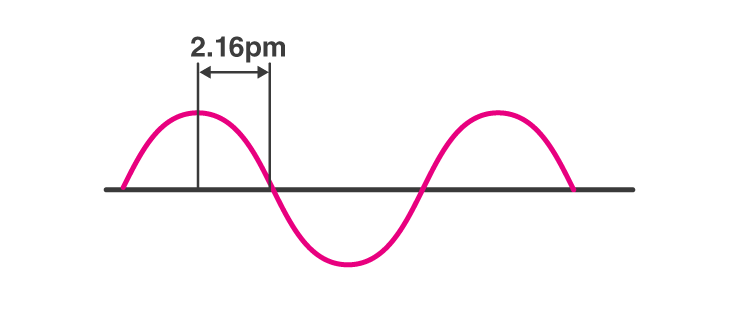Solution:

The wavelength can be defined as the distance between two alike successive points in a wave (usually b/w two maxima s i.e. peaks or two minima s i.e. troughs are shown in the fig.)

So, for the given hypothetical wave, wavelength λ = 4 * 2.16 pm

= 8.64 pm.

37. Chlorophyll present in green leaves of plants absorbs light at 4.620 × 1014 Hz. Calculate the wavelength of radiation in nanometer. Which part of the electromagnetic spectrum does it belong to?

Solution:

Relation b/w wavelength and frequency can be expressed as :

λ = c/ν, where c be the velocity of light and ν is the frequency of the radiation.

For the given problem λ = 3 x 108 ms-1 / 4.620 x 1014 Hz

= 0.6494 times10-6m-1

38. What is the difference between the terms orbit and orbital?

Solution:

The orbit stands for a definite circular path for the electrons to revolve around the nucleus. It represents the two-dimensional motion of the electrons around the nucleus, the orbital is not that well-defined path because it’s a region around the nucleus where the probability of finding an electron is maximum.

39. Table-tennis ball has a mass 10 g and a speed of 90 m/s. If speed can be measured within an accuracy of 4% what will be the uncertainty in speed and position?

Solution:

According to Heisenberg’s uncertainty principle :

“It is fundamentally impossible to determine accurately both the velocity and the position of a particle at the same time.

∆x. ∆p ≥ h/4π

From the given problem,

mass of the ball = 4 g and speed is = 90 m /s

hence,the uncertainity of speed is ∆v = 4/100 × 90 = 3.6 m/s

∆x is given by ∆x = h/4πm∆v

Hence , the uncertainity of postion is ∆x = 6.26 × 10-34 / 4 × 3.14 × 4× 3.6

= 1.46 x 10-33 m

40. The effect of the uncertainty principle is significant only for the motion of microscopic particles and is negligible for the macroscopic particles. Justify the statement with the help of a suitable example.

Solution:

The uncertainty principle is only significantly applicable for microscopic particles and not macroscopic particles this can be concluded from the measurement of uncertainty:

For example, if we take a particle or an object of mass 1 milligram i.e. 10-6 kg )

We calculate the,

∆x. ∆ν = 60626*10-34/ 4*3.14*10-6

= 10-28 m-2 s -1

The value we got is negligible and very insignificant for the uncertainty principle to apply to the particle.

41. The hydrogen atom has only one electron, so mutual repulsion between electrons is absent. However, in multielectron atoms mutual repulsion between the electrons is significant. How does this affect the energy of an electron in the orbitals of the same principal quantum number in multielectron atoms?

Solution:

The hydrogen atom has only one electron, so mutual repulsion between electrons is absent. However, in multielectron atoms mutual repulsion between the electrons is significant. How does this affect the energy of an electron in the orbitals of the same principal quantum number in multielectron atoms?

IV. Matching Type

In some of the following questions, one option of the left column maybe

Correlated to more than one option in the right column.

42. Match the following species with their corresponding ground state electronic

configuration.

 Atom / Ion (i) Cu (ii) Cu2+ (iii) Zn2+ (iv) Cr3+ Electronic configuration (a) 1s2 2s2 2p6 3s2 3p6 3d10 (b) 1s2 2s2 2p6 3s2 3p6 (c) 1s2 2s2 2p6 3s2 3p6 3d10 4s1 (d) 1s2 2s2 2p6 3s2 3p63d9 (e) 1s2 2s2 2p6 3s2 3p6 3d3

Solution:

(i) → c

(ii) →d

(iii) →a

(iv) →e

43. Match the quantum numbers with the information provided by these.

Quantum number Information provided

 (i) Principal quantum number (ii) Azimuthal quantum number (iii) Magnetic quantum number (iv) Spin quantum number (a) orientation of the orbital (b) energy and size of orbital (c)spin of an electron (d) shape of the orbital

Solution:

(i) → b

(ii) → d

(iii) → a

(iv) → c

44. Match the following

Rules Statements

 (i) Hund’s Rule (ii) Aufbau Principle (iii) Pauli Exclusion Principle (iv) Heisenberg’s Uncertainty Principle (a) No two electrons in an atom can have the same set of four quantum numbers. (b) Half-filled and filled orbitals have extra stability. (c) The pairing of electrons in the orbitals belonging to the same subshell does not take place until each orbital is singly occupied. (d) It is impossible to determine the exact position and exact the momentum of a subatomic particle simultaneously. (e) In the ground state of atoms, orbitals are filled in the order of their increasing energies.

Solution;

(i) → c

(ii) → e

(iii) → a

(iv) → d

45. Match the following

 (i) X-rays (ii) UV (iii) Long radio waves (iv) Microwave (a) ν = 100-0 104 Hz (b) ν = 1010Hz (c) ν = 1016 Hz (d) ν = 1018Hz

Solution:

(i)→ d

(ii)→ c

(iii) →a

(iv) →b

46. Match the following

 (i) Photon (ii) Electron (iii) ψ2 (iv) The principal quantum number n (a)Value is 4 for N shell (b)Probability density (c)Always a positive value (d)Exhibits both momentum and wavelength

Solution:

(i)→ d

(ii)→ d

(iii) →b, c

(iv)→ c, a

47. Match species are given in Column I with the electronic configuration given in Column II.

 Column I (i) Cr (ii) Fe2+ (iii) Ni2+ (iv) Cu (a) [Ar]3d84s0 (b) [Ar]3d104s1 (c) [Ar]3d64s0 (d) [Ar] 3d54s1 (e) [Ar]3d64s2

Solution:

(i) →d

(ii) →c

(iii) →a

(iv) →b

V. Assertion and Reason Type

In the following questions, a statement of Assertion (A) followed by a statement

Reason (R) is given. Choose the correct option out of the choices given

below each question.

48. . Assertion (A): All isotopes of a given element show the same type of chemical

behaviour.

Reason (R): The chemical properties of an atom are controlled by the

number of electrons in the atom.

(i) Both A and R are true and R is the correct explanation of A.

(ii) Both A and R are true but R is not the correct explanation of A.

(iii) A is true but R is false.

(iv) Both A and R are false.

Solution:

Option (i) is correct

49. Assertion (A): The black body is an ideal body that emits and absorbs radiations

of all frequencies.

Reason (R): The frequency of radiation emitted by a body goes from a lower

frequency to higher frequency with an increase in

temperature.

(i) Both A and R are true and R is the correct explanation of A.

(ii) Both A and R are true but R is not the explanation of A.

(iii) A is true and R is false.

(iv) Both A and R are false.

Solution:

50. Assertion (A): It is impossible to determine the exact position and exact

the momentum of an electron simultaneously.

Reason (R): The path of an electron in an atom is clearly defined.

(i) Both A and R are true and R is the correct explanation of A.

(ii) Both A and R are true and R is not the correct explanation of A.

(iii) A is true and R is false.

(iv) Both A and R are false.

Solution:

Option (iii) is correct

The structure of an atom is very important from the point of your future studies. It is one of the basic chapters that students need to learn to lay a good foundation for their future endeavours. In order to help students learn the structure of atoms thoroughly, BYJU’S brings you notes, exemplars, NCERT Solutions, worksheets, notes, question papers, sample papers, books, tips, and tricks. To get access to all the resources provided by us, register with the BYJU’S website, or download the BYJU’S learning app for a comprehensive learning opportunity.

## Frequently Asked Questions on NCERT Exemplar Solutions for Class 11 Chemistry Chapter 2

### What is the experimental evidence in support of the idea that electronic energies in an atom are quantized in the Chapter 2 of NCERT Exemplar Solutions for Class 11 Chemistry?

The bright-line spectrum shows that the energy levels in an atom are quantized. These lines are obtained as a result of electronic transitions between the energy and if the electronic energy levels were continuous and not quantized or discrete; the atomic spectra would have shown a continuous absorption(from lower to higher energy level transition) or emission (from higher to lower energy level transition.

### Can I get the NCERT Exemplar Solutions for Class 11 Chemistry Chapter 2 PDF online?

The NCERT Exemplar Solutions for Class 11 Chemistry Chapter 2 PDF is available on BYJU’S. These solutions are provided with a free download option which can be accessed by the students effortlessly. Students can download the PDF and refer to them while answering the textbook questions to get their doubts cleared instantly. The main advantage of using these solutions is, it can be used by the students anywhere and at any time without any difficulty.

### Does the NCERT Exemplar Solutions for Class 11 Chemistry Chapter 2 help students with their board exam preparation?

The NCERT Exemplar Solutions for Class 11 Chemistry Chapter 2 is very helpful for the students for various purposes. The expert faculty at BYJU’S make use of a simple and understandable language which makes it easy for the students while learning. These solutions provide students with a strong foundation of fundamental concepts which are important from the exam point of view. By using these solutions, students can save a lot of time in searching for the correct answer as per the CBSE syllabus and guidelines.